Next: Longitudinal Waves on a Up: Longitudinal Standing Waves Previous: Longitudinal Standing Waves

# Spring-Coupled Masses

Consider a mechanical system consisting of a linear array of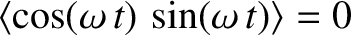identical masses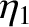that are free to slide in one dimension over a frictionless horizontal surface. Suppose that the masses are coupled to their immediate neighbors via identical light springs of unstreched length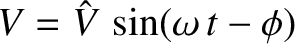, and force constant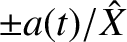. (Here, we employ the symbolto denote the spring force constant, rather than, becauseis already being used to denote wavenumber.) Let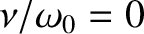measure distance along the array (from the left to the right). If the array is in its equilibrium state then the-coordinate of the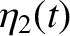th mass is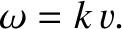, for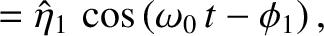. Consider longitudinal oscillations of the masses. Namely, oscillations for which the-coordinate of theth mass is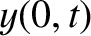(293)

where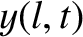represents longitudinal displacement from equilibrium. It is assumed that all of the displacements are relatively small: that is,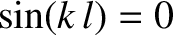, for.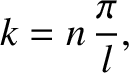Consider the equation of motion of theth mass. (See Figure 30.) The extensions of the springs to the immediate left and right of the mass are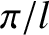and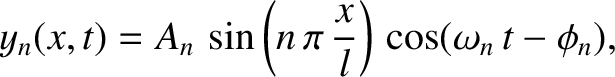, respectively. Thus, the-directed forces that these springs exert on the mass are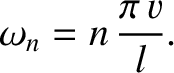and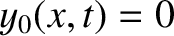, respectively. The mass's equation of motion therefore becomes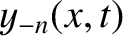(294)

where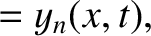. Because there is nothing special about theth mass, the preceding equation is assumed to hold for allmasses: that is, for. Equation (294), which governs the longitudinal oscillations of a linear array of spring-coupled masses, is analogous in form to Equation (232), which governs the transverse oscillations of a beaded string. This observation suggests that longitudinal and transverse waves in discrete dynamical systems (i.e., systems with a finite number of degrees of freedom) can be described using the same mathematical equations.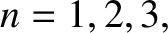We can interpret the quantities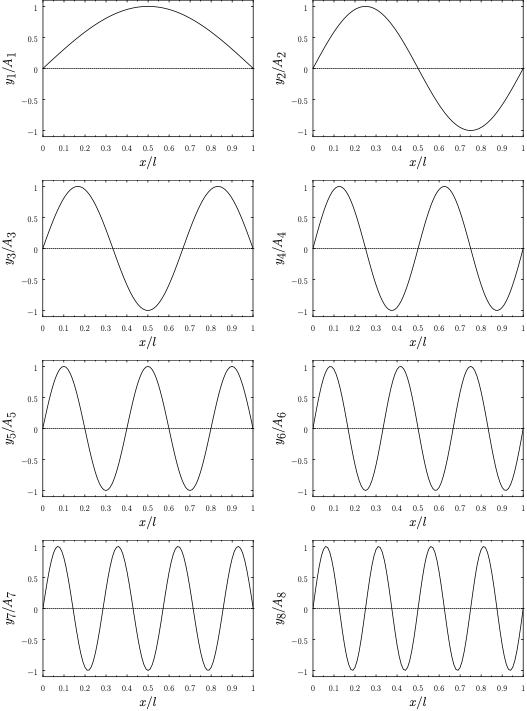and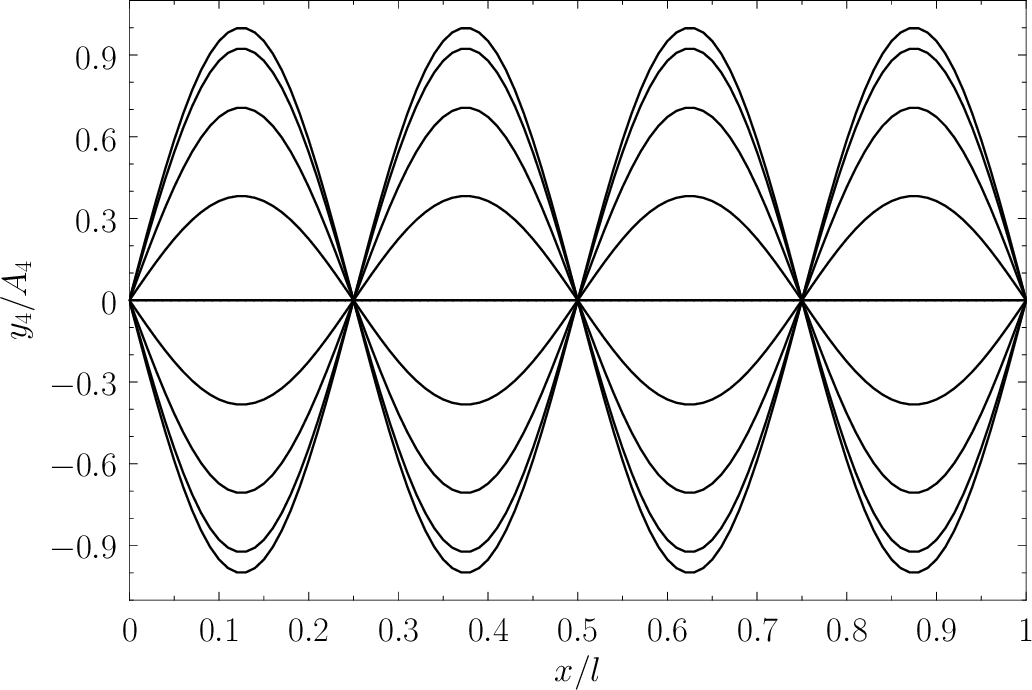, that appear in the equations of motion for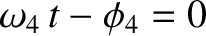and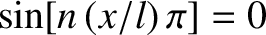, respectively, as the longitudinal displacements of the left and right extremities of springs attached to the outermost masses in such a manner as to form the left and right boundaries of the array. The respective equilibrium positions of these extremities are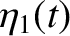and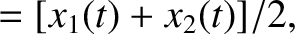. The end displacements,and, must be prescribed, otherwise Equations (294) do not constitute a complete set of equations. In other words, there are more unknowns than equations. The particular choice ofanddepends on the nature of the physical boundary conditions at the two ends of the array. Suppose that the left extremity of the leftmost spring is anchored in an immovable wall. This implies that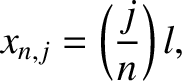: that is, the left extremity of the spring cannot move. Suppose, on the other hand, that the left extremity of the leftmost spring is not attached to anything. In this case, there is no reason for the spring to become extended, which implies that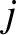. In other words, if the left end of the array is fixed (i.e., attached to an immovable object) then, and if the left end is free (i.e., not attached to anything) then. Likewise, if the right end of the array is fixed then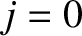, and if the right end is free then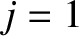.

Suppose, for the sake of argument, that the left end of the array is free, and the right end is fixed. It follows that, and. Let us search for normal modes of the general form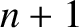(295)

where,,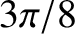, andare constants. The preceding expression automatically satisfies the boundary condition. This follows becauseand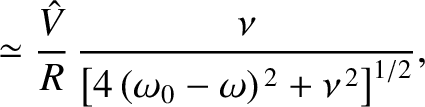, and, consequently,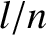. The other boundary condition,, is satisfied provided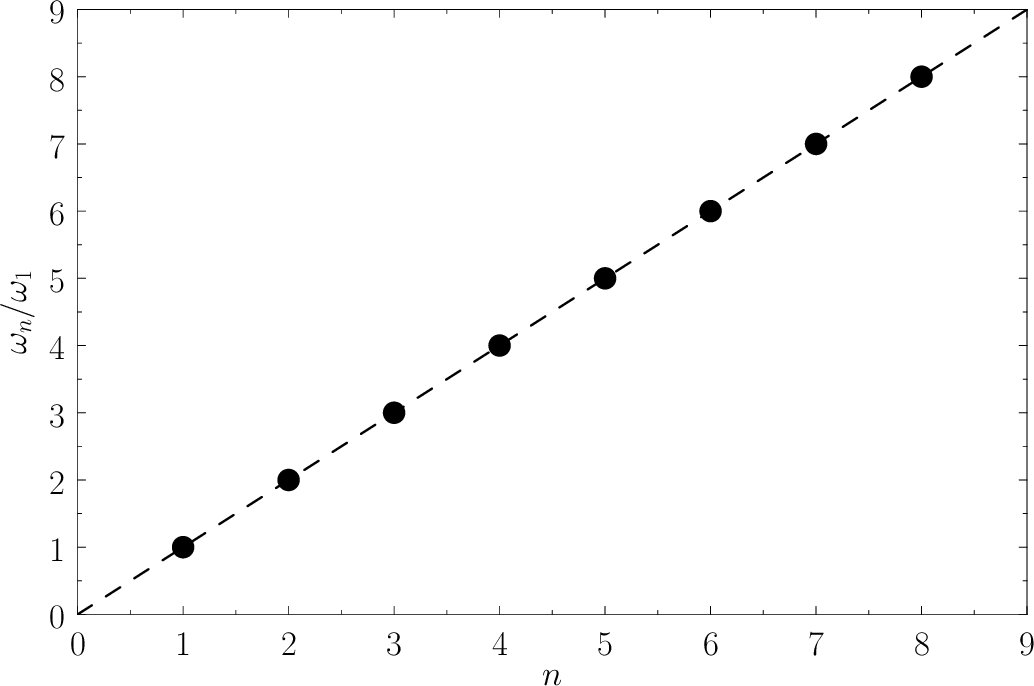(296)

which yields [cf., Equation (242)]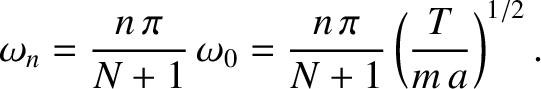(297)

where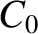is an integer. As before, the imposition of the boundary conditions causes a quantization of the possible mode wavenumbers. (See Section 5.1.) Finally, substitution of Equation (295) into Equation (294) gives the dispersion relation [cf., Equation (240)]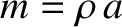(298)

It follows, from the preceding analysis, that the longitudinal normal modes of a linear array of spring-coupled masses, the left end of which is free, and the right end fixed, are associated with the following characteristic displacement patterns,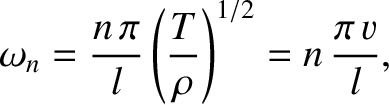(299)

where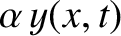(300)

and the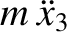and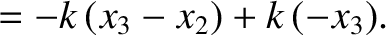are arbitrary constants determined by the initial conditions. Here, the integerindexes the masses, and the mode numberindexes the normal modes. It can be demonstrated that there are onlyunique normal modes, corresponding to mode numbers in the range 1 to.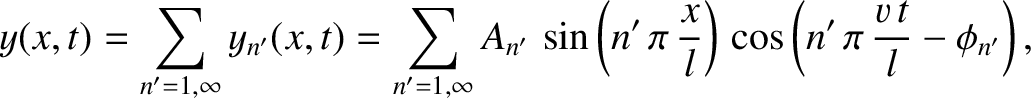Figures 31 and 295 display the normal modes and normal frequencies of a linear array of eight spring-coupled masses, the left end of which is free, and the right end fixed. The data shown in these figures is obtained from Equations (299) and (300), respectively, with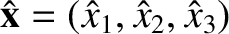. The modes in Figure 31 are all plotted at the instances in time when they attain their maximum amplitudes: namely, when. It can be seen that normal modes with small wavenumbers--that is,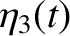, so that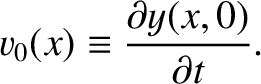--have displacements that vary in a fairly smooth sinusoidal manner from mass to mass, and oscillations frequencies that increase approximately linearly with increasing wavenumber. On the other hand, normal modes with large wavenumbers--that is,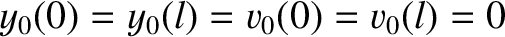, so that--have displacements that exhibit large variations from mass to mass, and oscillation frequencies that do not depend linearly on wavenumber. We conclude that the longitudinal normal modes of an array of spring-coupled masses have analogous properties to the transverse normal modes of a beaded string. (See Section 5.1.)

The dynamical system pictured in Figure 30 can be used to model the effect of a planar sound wave (i.e., a longitudinal oscillation in position that is periodic in space) on a crystal lattice. In this application, the masses represent parallel planes of atoms, the springs represent the interatomic forces acting between these planes, and the longitudinal oscillations represent the sound wave. Of course, a macroscopic crystal contains a great many atomic planes, so we would expectto be very large. However, according to Equations (297) and (300), no matter how largebecomes,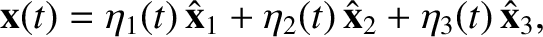cannot exceed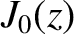(becausecannot exceed), and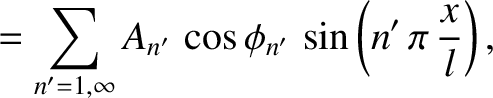cannot exceed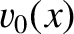. In other words, there is a minimum wavelength that a sound wave in a crystal lattice can have, which turns out to be twice the interplane spacing, and a corresponding maximum oscillation frequency. For waves whose wavelengths are much greater than the interplane spacing (i.e.,), the dispersion relation (298) reduces to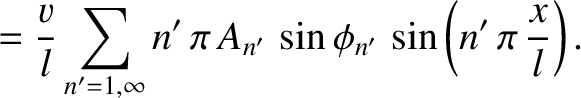(301)

where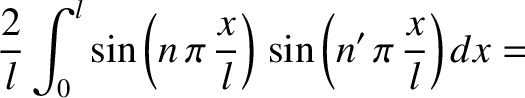is a constant that has the dimensions of velocity. It seems plausible that Equation (301) is the dispersion relation for sound waves in a continuous elastic medium. Let us investigate such waves.Next: Longitudinal Waves on a Up: Longitudinal Standing Waves Previous: Longitudinal Standing Waves
Richard Fitzpatrick 2013-04-08